# Axis support in WinUI Chart

5 Apr 202224 minutes to read

`ChartAxis` is used to locate a data point inside the chart area. Charts typically have two axes that are used to measure and categorize data: a vertical (Y) axis, and a horizontal (X) axis.

Vertical(Y) axis always uses numerical scale. Horizontal(X) axis supports the following types of scale:

• Category
• Numeric
• Date time

The following are the API’s in ChartAxis:

The following topics explain in detail about the axis and its parts:

In `ChartAxis`, you can define any object as header using `Header` property. The following code example demonstrates how to define a header in primary and secondary axes.

``````<syncfusion:SfChart.PrimaryAxis>

</syncfusion:SfChart.PrimaryAxis>

<syncfusion:SfChart.SecondaryAxis>

</syncfusion:NumericalAxis>

</syncfusion:SfChart.SecondaryAxis>``````
``````chart.PrimaryAxis = new CategoryAxis() { Header = "Metals" };

chart.SecondaryAxis = new NumericalAxis() { Header = "Values(In Tonnes)" };``````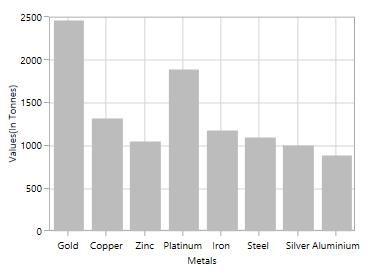The default appearance of the header can be customized using the `HeaderTemplate` property. The following code sample demonstrates the header customization.

``````<syncfusion:SfChart x:Name="chart">

<syncfusion:SfChart.Resources>

<TextBlock Text="Demands" FontSize="12" FontStyle="Italic"

FontWeight="Bold" Margin="3"/>

</Border>

</DataTemplate>

<TextBlock Text="Values(In Tonnes)" FontSize="12" FontStyle="Italic"

FontWeight="Bold" Margin="3"/>

</Border>

</DataTemplate>

</syncfusion:SfChart.Resources>

<syncfusion:SfChart.PrimaryAxis>

</syncfusion:SfChart.PrimaryAxis>

<syncfusion:SfChart.SecondaryAxis>

</syncfusion:SfChart.SecondaryAxis>

</syncfusion:SfChart>``````
``````chart.PrimaryAxis = new CategoryAxis()
{

};

chart.SecondaryAxis = new NumericalAxis()
{

};``````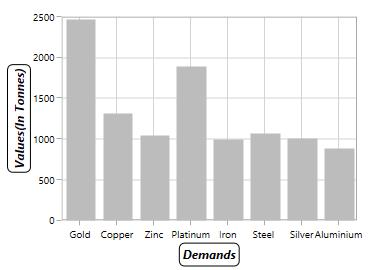`HeaderStyle` property is used to provide style for the axis header. The following code example explains how to customize the header style.

``````<syncfusion:SfChart.PrimaryAxis>

<syncfusion:LabelStyle FontFamily="Algerian" FontSize="13" Foreground="Black">

</syncfusion:LabelStyle>

</syncfusion:CategoryAxis>

</syncfusion:SfChart.PrimaryAxis>

<syncfusion:SfChart.SecondaryAxis>

<syncfusion:LabelStyle FontFamily="Algerian" FontSize="13" Foreground="Black">

</syncfusion:LabelStyle>

</syncfusion:NumericalAxis>

</syncfusion:SfChart.SecondaryAxis>``````
``````LabelStyle style = new LabelStyle()
{

FontFamily = new FontFamily("Algerian"),

FontSize = 13,

Foreground = new SolidColorBrush(Colors.Black)

};

chart.PrimaryAxis = new CategoryAxis()
{

LabelStyle = style

};

chart.SecondaryAxis = new NumericalAxis()
{

LabelStyle = style

};``````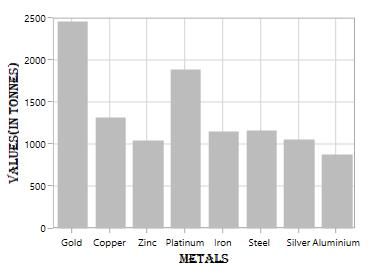## Axis Labels

Labels will be generated by the range and the values binded to `XBindingPath` or `YBindingPath` properties.

Positioning the Labels

The `LabelPosition` property is used to position the axis label either inside or outside the chart plotting area. By default, LabelPosition is `Outside`.

Inside

``````<syncfusion:SfChart.PrimaryAxis>

<syncfusion:NumericalAxis LabelPosition="Inside">

</syncfusion:NumericalAxis>

</syncfusion:SfChart.PrimaryAxis>``````
``````chart.PrimaryAxis = new NumericalAxis()
{

LabelPosition = AxisElementPosition.Inside

};``````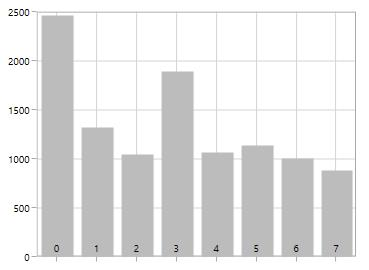LabelRotationAngle

`LabelRotationAngle` property allows you to define the angle for the label content. The following code example illustrates the `LabelRotationAngle`.

``````<syncfusion:SfChart.PrimaryAxis>

<syncfusion:DateTimeAxis LabelRotationAngle="90">

</syncfusion:DateTimeAxis>

</syncfusion:SfChart.PrimaryAxis>``````
``````chart.PrimaryAxis = new DateTimeAxis()
{

LabelRotationAngle = 90

};``````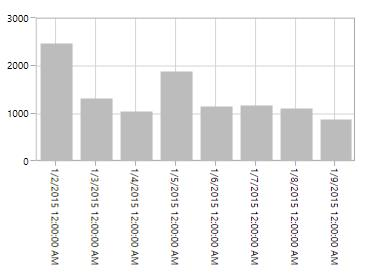Custom Labels

`SfChart` allows the user to define the labels for the axis. For defining the axis label, you have to set the `Content` and `Position` properties. You can define the labels using `CustomLabels` property as shown in the following code sample.

``````<syncfusion:SfChart.PrimaryAxis>

<syncfusion:CategoryAxis >

<syncfusion:CategoryAxis.CustomLabels>

<syncfusion:ChartAxisLabel Position="0" Content="0-1"/>

<syncfusion:ChartAxisLabel Position="1" Content="1-2"/>

<syncfusion:ChartAxisLabel Position="2" Content="2-3"/>

<syncfusion:ChartAxisLabel Position="3" Content="3-4"/>

<syncfusion:ChartAxisLabel Position="4" Content="4-5"/>

<syncfusion:ChartAxisLabel Position="5" Content="5-5"/>

</syncfusion:CategoryAxis.CustomLabels>

</syncfusion:CategoryAxis>

</syncfusion:SfChart.PrimaryAxis>``````
``````CategoryAxis axis = new CategoryAxis();

axis.CustomLabels.Add(new ChartAxisLabel() { Position = 0, Content = "0-1" });

axis.CustomLabels.Add(new ChartAxisLabel() { Position = 1, Content = "1-2" });

axis.CustomLabels.Add(new ChartAxisLabel() { Position = 2, Content = "2-3" });

axis.CustomLabels.Add(new ChartAxisLabel() { Position = 3, Content = "3-4" });

axis.CustomLabels.Add(new ChartAxisLabel() { Position = 4, Content = "4-5" });

axis.CustomLabels.Add(new ChartAxisLabel() { Position = 5, Content = "5-5" });

chart.PrimaryAxis = axis;``````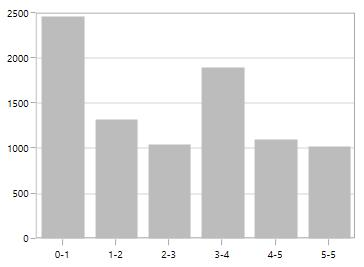You can also directly bind the collection of labels to the `LabelSource` property for defining custom labels. The following code example demonstrates how to define the label collection in code behind and then bind the property in XAML page.

``````<syncfusion:SfChart.PrimaryAxis>

<syncfusion:CategoryAxis LabelSource="{Binding Labels}" ContentPath="Content" PositionPath="Position">

</syncfusion:CategoryAxis>

</syncfusion:SfChart.PrimaryAxis>``````
``````chart.PrimaryAxis = new CategoryAxis()
{

ContentPath = "Content",

PositionPath = "Position",

LabelSource = Labels

};

public List<LabelItem> Labels { get; set; }

Labels = new List<LabelItem>
{

new LabelItem() {Position =  0, Content = "0-1"},

new LabelItem() {Position = 1, Content = "1-2"},

new LabelItem() {Position = 2, Content = "2-3"},

new LabelItem() {Position = 3, Content = "3-4"},

new LabelItem() {Position = 4, Content = "4-5"},

new LabelItem() {Position = 5, Content = "5-6"},

new LabelItem() {Position = 6, Content = "6-7"},

new LabelItem() {Position = 7, Content = "7-8"},

};

public class LabelItem
{

public string Content { get; set; }

public int Position { get; set; }

}``````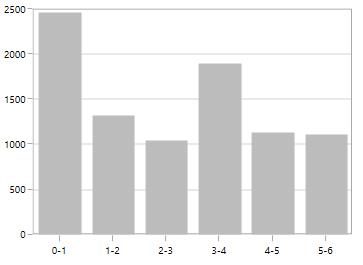Label Formatting

Axis labels can be formatted using predefined formatting types based on the axis types.

DateTimeAxis

``````<syncfusion:SfChart.PrimaryAxis>

<syncfusion:DateTimeAxis LabelFormat="MMM/dd" FontSize="12">

</syncfusion:DateTimeAxis>

</syncfusion:SfChart.PrimaryAxis>``````
``````chart.PrimaryAxis = new DateTimeAxis()
{

LabelFormat = "MM/dd",

FontSize = 12

};``````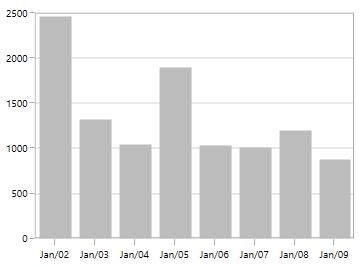NumericalAxis

``````<syncfusion:SfChart.SecondaryAxis>

<syncfusion:NumericalAxis LabelFormat="0.00"/>

</syncfusion:SfChart.SecondaryAxis>``````
``````chart.PrimaryAxis = new NumericalAxis()
{

LabelFormat = "0.00",

};``````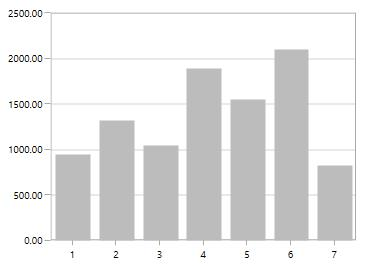LabelTemplate

The `LabelTemplate` property allows you to define the appearance for the axis labels. The following code example illustrates the `LabelTemplate` property.

``````<syncfusion:SfChart x:Name="chart">

<syncfusion:SfChart.Resources>

<DataTemplate x:Key="labelTemplate">

<TextBlock Text="{Binding Content}" Foreground="White"

FontStyle="Normal" FontSize="10"

FontWeight="Bold" Margin="3"/>

<Border.Effect>

Color="LightGray" Opacity="0.25"

</Border.Effect>

</Border>

</DataTemplate>

</syncfusion:SfChart.Resources>

<syncfusion:SfChart.PrimaryAxis>

<syncfusion:CategoryAxis LabelTemplate="{StaticResource labelTemplate}"/>

</syncfusion:SfChart.PrimaryAxis>

</syncfusion:SfChart>``````
``````chart.PrimaryAxis = new CategoryAxis()
{

LabelTemplate = chart.Resources["labelTemplate"] as DataTemplate

};``````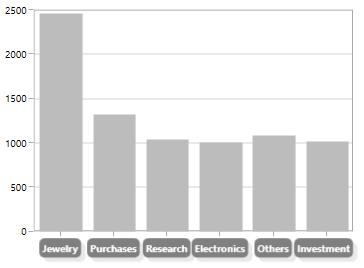LabelExtent

This property allows us to set the distance between the axis header and the axis using the `LabelExtent` property. The following code sample defines the `LabelExtent` property.

``````<syncfusion:SfChart.PrimaryAxis>

</syncfusion:CategoryAxis>``````
``````chart.PrimaryAxis = new NumericalAxis()
{

LabelExtent = 50

};``````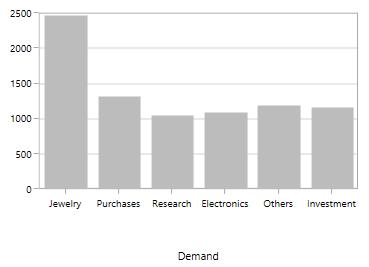Smart Axis Labels

When there are more number of axis labels, they may overlap with each other. `SfChart` provides support to handle the overlapping labels using the `LabelIntersectAction` property. By default, the `LabelIntersectAction` value is `Hide`.

The following are the options for intersecting action.

• None
• Hide
• MultipleRows
• Auto

None

None option is used to display all the label, even if it intersects. The following code sample demonstrates the `LabelIntersectAction` as None option.

``````<syncfusion:SfChart.PrimaryAxis>

<syncfusion:CategoryAxis LabelIntersectAction="None"/>

</syncfusion:SfChart.PrimaryAxis>``````
``````chart.PrimaryAxis = new CategoryAxis()
{

LabelIntersectAction = AxisLabelIntersectAction.None

};``````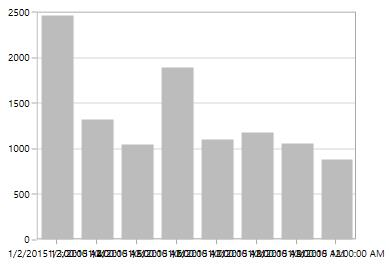Hide

Hide option is used to hide the labels if it intersects. You can define the hide as shown in the below code snippet.

``````<syncfusion:SfChart.PrimaryAxis>

<syncfusion:DateTimeAxis LabelIntersectAction="Hide"/>

</syncfusion:SfChart.PrimaryAxis>``````
``````chart.PrimaryAxis = new DateTimeAxis()
{

LabelIntersectAction = AxisLabelIntersectAction.Hide

};``````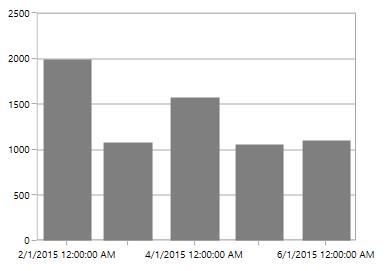MultipleRows

This option is used to move the labels to next row if it intersects. The following code demonstrates the MultipleRows option in `LabelIntersectAction`.

``````<syncfusion:SfChart.PrimaryAxis>

<syncfusion:DateTimeAxis LabelIntersectAction="MultipleRows">

</syncfusion:DateTimeAxis>

</syncfusion:SfChart.PrimaryAxis>``````
``````chart.PrimaryAxis = new DateTimeAxis()
{

LabelIntersectAction = AxisLabelIntersectAction.MultipleRows

};``````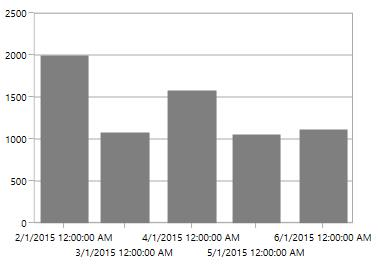Auto

This option in `LabelIntersectAction` property is used to rotate the labels if they intersects. The following code sample and image demonstrate the rotate option in the `LabelIntersectAction` property.

``````<syncfusion:CategoryAxis LabelIntersectAction="Auto">

</syncfusion:CategoryAxis>``````
``````chart.PrimaryAxis = new CategoryAxis()
{

LabelIntersectAction = AxisLabelIntersectAction.Auto

};``````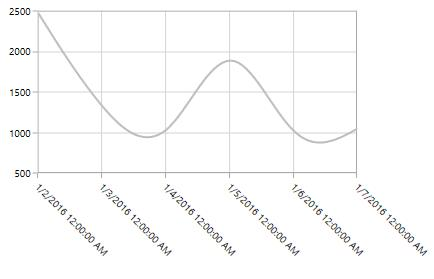EdgeLabelsDrawingMode

`SfChart` provides support to customize the position of the edge labels in axis using the `EdgeLabelsDrawingMode` property. `EdgeLabelsDrawingMode` property default value is `Center`.

The following are the customizing options in `EdgeLabelsDrawingMode`.

• Center - Positions the label with tick line as center.
• Fit - Position the gridline inside based on the edge label size.
• Hide - Hides the edge labels.
• Shift - Shifts the edge labels to the left or right so that it comes inside the chart area.

Center

``````<syncfusion:SfChart.PrimaryAxis>

<syncfusion:DateTimeAxis EdgeLabelsDrawingMode="Center">

</syncfusion:DateTimeAxis>

</syncfusion:SfChart.PrimaryAxis>``````
``````chart.PrimaryAxis = new DateTimeAxis()
{

EdgeLabelsDrawingMode = EdgeLabelsDrawingMode.Center

};``````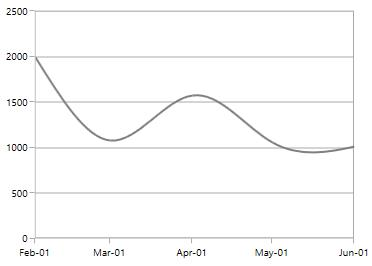Shift

``````<syncfusion:SfChart.PrimaryAxis>

<syncfusion:DateTimeAxis EdgeLabelsDrawingMode="Shift">

</syncfusion:DateTimeAxis>

</syncfusion:SfChart.PrimaryAxis>``````
``````chart.PrimaryAxis = new DateTimeAxis()
{

EdgeLabelsDrawingMode = EdgeLabelsDrawingMode.Shift

};``````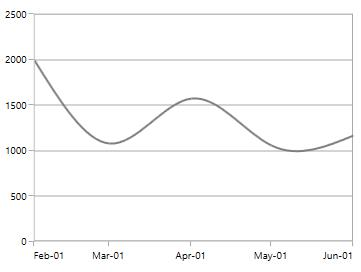Hide

``````<syncfusion:SfChart.PrimaryAxis>

<syncfusion:DateTimeAxis EdgeLabelsDrawingMode="Hide">

</syncfusion:DateTimeAxis>

</syncfusion:SfChart.PrimaryAxis>``````
``````chart.PrimaryAxis = new DateTimeAxis()
{

EdgeLabelsDrawingMode = EdgeLabelsDrawingMode.Hide

};``````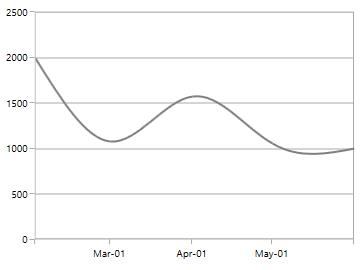Fit

``````<syncfusion:SfChart.PrimaryAxis>

<syncfusion:DateTimeAxis EdgeLabelsDrawingMode="Fit">

</syncfusion:DateTimeAxis>

</syncfusion:SfChart.PrimaryAxis>``````
``````chart.PrimaryAxis = new DateTimeAxis()
{

EdgeLabelsDrawingMode = EdgeLabelsDrawingMode.Fit

};``````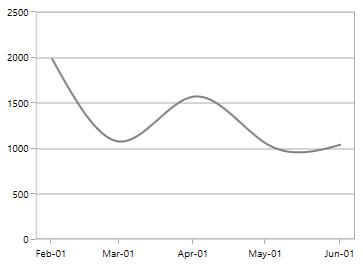EdgeLabelsVisibilityMode

The visibility of the extreme labels of the axis can be controlled using `EdgeLabelsVisibilityMode` property. By default, the `Default` option in `EdgeLabelsVisibilityMode` is set, which displays the edge label based on auto interval calculations. The following image depicts the default option in `EdgeLabelsVisibilityMode` while zooming.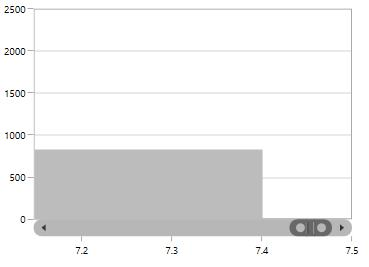Always Visible

AlwaysVisible option in `EdgeLabelsVisibilityMode` is used to view the edge labels even while performing zooming.

The following code example and image demonstrate the `AlwaysVisible` option while zooming.

``````<syncfusion:SfChart.PrimaryAxis>

<syncfusion:NumericalAxis EdgeLabelsVisibilityMode="AlwaysVisible">

</syncfusion:NumericalAxis>

</syncfusion:SfChart.PrimaryAxis>``````
``````chart.PrimaryAxis = new NumericalAxis()
{

EdgeLabelsVisibilityMode = EdgeLabelsVisibilityMode.AlwaysVisible

};``````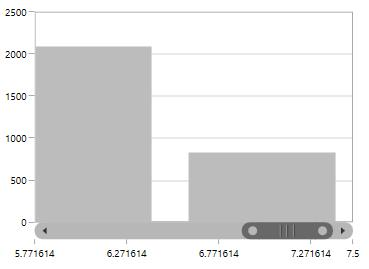Visible

Visible option is used to display the edge labels (first and last label) irrespective of the auto-interval calculation until zooming (i.e., in normal state).

``````<syncfusion:SfChart.PrimaryAxis>

<syncfusion:NumericalAxis EdgeLabelsVisibilityMode="Visible">

</syncfusion:NumericalAxis>

</syncfusion:SfChart.PrimaryAxis>``````
``````chart.PrimaryAxis = new NumericalAxis()
{

EdgeLabelsVisibilityMode = EdgeLabelsVisibilityMode.Visible

};``````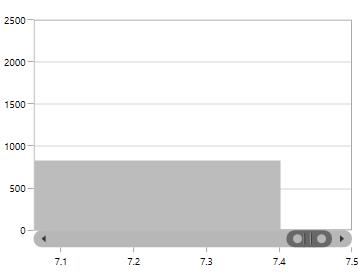## Grid lines

By default, gridlines are automatically added to the `ChartAxis` in its defined intervals. `SfChart` supports customization of gridline. The visibility of the gridlines can be controlled using the `ShowGridLines` property.

The following code example illustrates the `ShowGridLines` property as false in the primary axis.

``````<syncfusion:SfChart.PrimaryAxis>

<syncfusion:NumericalAxis ShowGridLines="False">

</syncfusion:NumericalAxis>

</syncfusion:SfChart.PrimaryAxis>``````
``````chart.PrimaryAxis = new NumericalAxis()
{

ShowGridLines = false

};``````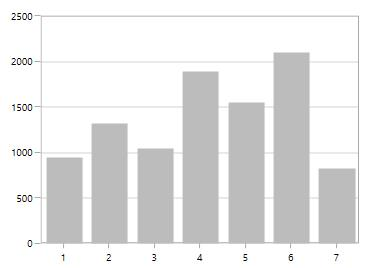Style can also be applied to Major and Minor gridlines using the `MajorGridLineStyle` and `MinorGridLineStyle` properties.

MajorGridLineStyle

``````<syncfusion:SfChart x:Name="chart">

<syncfusion:SfChart.Resources>

<Style TargetType="Line" x:Key="lineStyle">

<Setter Property="StrokeThickness" Value="2"/>

<Setter Property="Stroke" Value="Black"/>

<Setter Property="StrokeDashArray" Value="3,3"/>

</Style>

</syncfusion:SfChart.Resources>

<syncfusion:SfChart.PrimaryAxis>

<syncfusion:NumericalAxis MajorGridLineStyle="{StaticResource lineStyle}"/>

</syncfusion:SfChart.PrimaryAxis>

</syncfusion:SfChart>``````
``````chart.PrimaryAxis = new NumericalAxis()
{

MajorGridLineStyle = chart.Resources["lineStyle"] as Style

};``````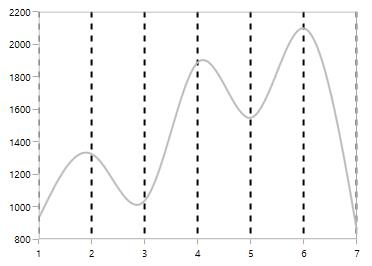MinorGridLineStyle

Minor gridlines will be added automatically when the small tick lines is defined inside the chart area.

``````<syncfusion:SfChart x:Name="chart">

<syncfusion:SfChart.Resources>

<Style TargetType="Line" x:Key="lineStyle">

<Setter Property="StrokeThickness" Value="1"/>

<Setter Property="Stroke" Value="DarkGray"/>

<Setter Property="StrokeDashArray" Value="3,3"/>

</Style>

</syncfusion:SfChart.Resources>

<syncfusion:SfChart.SecondaryAxis>

<syncfusion:NumericalAxis SmallTicksPerInterval="3"

MinorGridLineStyle="{StaticResource lineStyle}"/>

</syncfusion:SfChart.SecondaryAxis>

</syncfusion:SfChart>``````
``````chart.PrimaryAxis = new NumericalAxis()
{

SmallTicksPerInterval = 3,

MinorGridLineStyle = chart.Resources["lineStyle"] as Style

};``````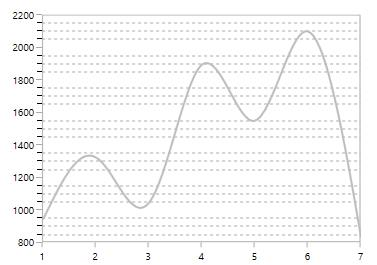## Tick lines

Tick lines are the small lines, which is drawn on the axis line representing the axis labels. Tick lines will be drawn outside of the axis by default.

TickLineSize

Tick lines height can be customized using the `TickLineSize` property as shown in the following code sample.

``````<syncfusion:SfChart.PrimaryAxis>

<syncfusion:NumericalAxis TickLineSize="10">

</syncfusion:NumericalAxis>

</syncfusion:SfChart.PrimaryAxis>``````
``````chart.PrimaryAxis = new NumericalAxis()
{

TickLineSize = 10

};``````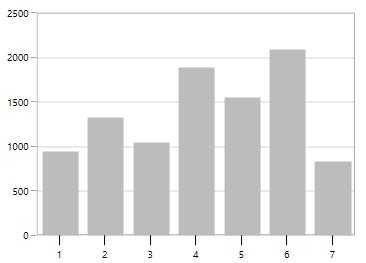Positioning the Major Tick Lines

Tick lines can be positioned inside or outside of the chart area using the `TickLinesPosition` property. By default, the tick lines will be positioned outside of the chart area. The following code example demonstrates how to position the tick lines inside of the chart area.

``````<syncfusion:SfChart.PrimaryAxis>

<syncfusion:NumericalAxis TickLinesPosition="Inside">

</syncfusion:NumericalAxis>

</syncfusion:SfChart.PrimaryAxis>``````
``````chart.PrimaryAxis = new NumericalAxis()
{

TickLinesPosition = AxisElementPosition.Inside

};``````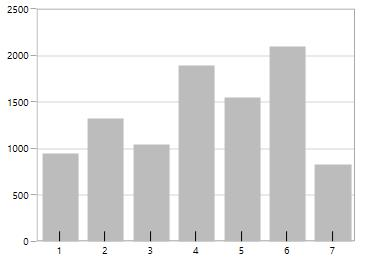Customization

Style can be applied to major tick lines using the `MajorTickLineStyle` property. The following code sample demonstrates the styling of major tick lines.

``````<syncfusion:SfChart x:Name="chart">

<syncfusion:SfChart.Resources>

<Style TargetType="Line" x:Name="lineStyle">

<Setter Property="StrokeThickness" Value="1"/>

<Setter Property="Stroke" Value="Black"/>

<Setter Property="StrokeDashArray" Value="3,3"/>

</Style>

</syncfusion:SfChart.Resources>

<syncfusion:SfChart.SecondaryAxis>

<syncfusion:NumericalAxis TickLineSize="10"

MajorTickLineStyle="{StaticResource lineStyle}"/>

</syncfusion:SfChart.SecondaryAxis>

</syncfusion:SfChart>``````
``````chart.PrimaryAxis = new NumericalAxis()
{

TickLineSize = 10,

MajorTickLineStyle = chart.Resources["lineStyle"] as Style

};``````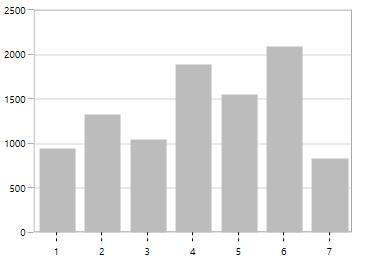MinorTickLines

Minor tick lines can be added by defining the `SmallTicksPerInterval` property. This property will add the tick lines to every interval.

The following code example demonstrates the small ticks set for every interval.

``````<syncfusion:SfChart.PrimaryAxis>

<syncfusion:NumericalAxis Interval="1" SmallTicksPerInterval="4" >

</syncfusion:NumericalAxis>

</syncfusion:SfChart.PrimaryAxis>``````
``````chart.PrimaryAxis = new NumericalAxis()
{

Interval = 1,

SmallTicksPerInterval = 4

};``````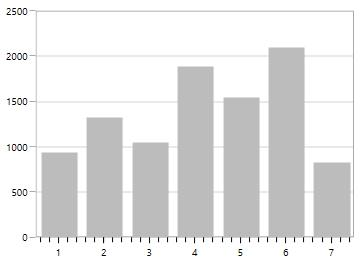Positioning the minor tick lines

Minor tick lines can be positioned inside or outside using the `SmallTickLinesPosition` property. By default, the minor tick lines will be positioned outside.

The following code example demonstrates how to position the minor tick lines inside the chart area.

``````<syncfusion:SfChart.PrimaryAxis>

<syncfusion:NumericalAxis SmallTicksPerInterval="2" SmallTickLinesPosition="Inside">

</syncfusion:NumericalAxis>

</syncfusion:SfChart.PrimaryAxis>``````
``````chart.PrimaryAxis = new NumericalAxis()
{

SmallTicksPerInterval = 2,

SmallTickLinesPosition = AxisElementPosition.Inside

};``````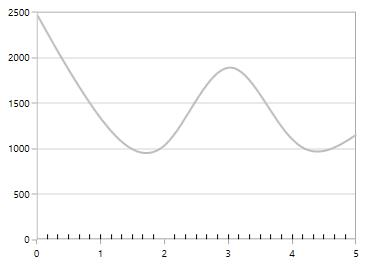Customization of Minor Ticklines

The height of the minor tick lines can be customized using the `SmallTickLineSize` property as shown in the following code sample.

``````<syncfusion:SfChart.PrimaryAxis>

<syncfusion:NumericalAxis Interval="1" SmallTicksPerInterval="3" SmallTickLineSize="10">

</syncfusion:NumericalAxis>

</syncfusion:SfChart.PrimaryAxis>``````
``````chart.PrimaryAxis = new NumericalAxis()
{

Interval = 1,

SmallTicksPerInterval = 3,

SmallTickLineSize = 10

};``````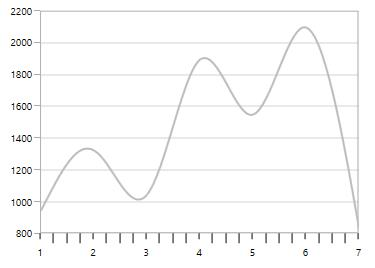Styling customization of minor tick lines can be defined using the `MinorTickLineStyle` property. The following code example and image demonstrates the style for minor tick lines.

``````<syncfusion:SfChart x:Name="chart">

<syncfusion:SfChart.Resources>

<Style TargetType="Line" x:Name="lineStyle" >

<Setter Property="StrokeThickness" Value="0.5"/>

<Setter Property="Stroke" Value="Black"/>

</Style>

</syncfusion:SfChart.Resources>

<syncfusion:SfChart.SecondaryAxis>

<syncfusion:NumericalAxis FontSize="12"  Interval="1"

SmallTicksPerInterval="3"

TickLineSize="10" SmallTickLineSize="5"

MinorTickLineStyle="{StaticResource lineStyle}"/>

</syncfusion:SfChart.SecondaryAxis>

</syncfusion:SfChart>``````
``````chart.PrimaryAxis = new NumericalAxis()
{

Interval = 1,

SmallTicksPerInterval = 3,

TickLineSize = 10,

SmallTickLineSize = 5

MinorTickLineStyle = chart.Resources["lineStyle"] as Style

};``````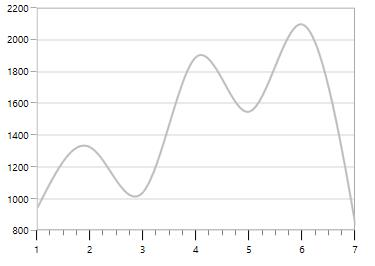NOTE

For category axis, small tick lines is not applicable since it is rendered based on index positions.

## AxisLine

`SfChart` provides support to customize the style of the axis line by defining the `AxisLineStyle` property as shown in the following code sample.

``````<syncfusion:SfChart.PrimaryAxis>

<syncfusion:NumericalAxis Interval="1">

<syncfusion:NumericalAxis.AxisLineStyle>

<Style TargetType="Line"  >

<Setter Property="StrokeThickness" Value="2"/>

<Setter Property="Stroke" Value="Red"/>

<Setter Property="StrokeDashArray" Value="2,2"/>

</Style>

</syncfusion:NumericalAxis.AxisLineStyle>

</syncfusion:NumericalAxis>

</syncfusion:SfChart.PrimaryAxis>``````
``````chart.PrimaryAxis = new NumericalAxis()
{

Interval = 1,

AxisLineStyle = chart.Resources["lineStyle"] as Style

};``````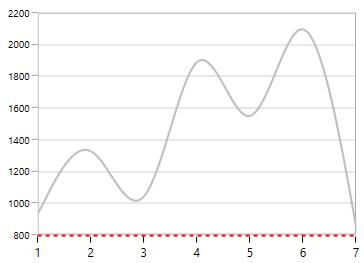Applying Padding to the Axis line

The padding to the axis line is defined using the `AxisLineOffset` property. The following code example demonstrates how to set `AxisLineOffset` for the x-axis.

``````<syncfusion:SfChart.PrimaryAxis>

<syncfusion:NumericalAxis AxisLineOffset="20">

</syncfusion:NumericalAxis>

</syncfusion:SfChart.PrimaryAxis>``````
``````chart.PrimaryAxis = new NumericalAxis()
{

AxisLineOffset = 20

};``````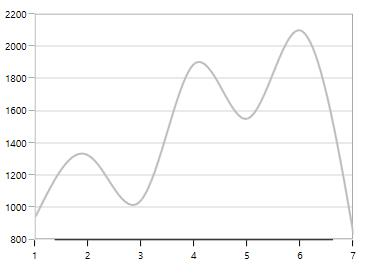## Types of Axis

`ChartAxis` supports the following types:

• NumericalAxis
• CategoryAxis
• DateTimeAxis

You can choose any type of `ChartAxis` such as`DateTimeAxis`, `NumericalAxis`, or `CategoryAxis` depending on the value type.

### NumericalAxis

`NumericalAxis` is used to plot numerical values to the chart. `NumericalAxis` can be defined for both `PrimaryAxis` and `SecondaryAxis`. The following code sample shows how to define the `NumericalAxis`.

``````<syncfusion:SfChart.PrimaryAxis>

<syncfusion:NumericalAxis>

</syncfusion:NumericalAxis>

</syncfusion:SfChart.PrimaryAxis>

<syncfusion:SfChart.SecondaryAxis>

<syncfusion:NumericalAxis>

</syncfusion:NumericalAxis>

</syncfusion:SfChart.SecondaryAxis>``````
``````chart.PrimaryAxis = new NumericalAxis();

chart.SecondaryAxis = new NumericalAxis();``````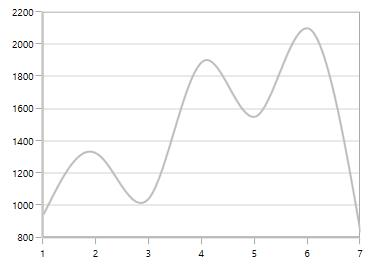Customizing the NumericalAxis Range

The `Maximum` property is used for setting the maximum value for the axis range and `Minimum` property is used for setting the minimum value for the axis range.

``````<syncfusion:SfChart.SecondaryAxis>

<syncfusion:NumericalAxis Maximum="2750" Minimum="250" Interval="250">

</syncfusion:NumericalAxis>

</syncfusion:SfChart.SecondaryAxis>``````
``````chart.SecondaryAxis = new NumericalAxis()
{

Maximum = 2750,

Minimum = 250,

Interval = 250

};``````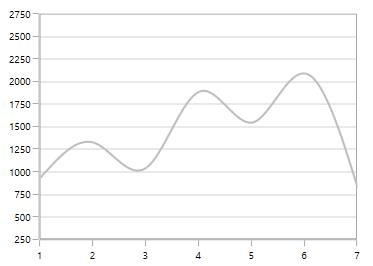NOTE

If minimum or maximum value is set, the other value is calculated by default internally.

StartRangeFromZero

`NumericalAxis` will calculate the range based on the data points bound to the axis. To start the range from zero, set the `StartRangeFromZero` property to True. The following code example demonstrates the NumericalAxis range starting from zero.

``````<syncfusion:SfChart.SecondaryAxis>

<syncfusion:NumericalAxis StartRangeFromZero="True">

</syncfusion:NumericalAxis>

</syncfusion:SfChart.SecondaryAxis>``````
``````chart.SecondaryAxis = new NumericalAxis()
{

StartRangeFromZero = true

};``````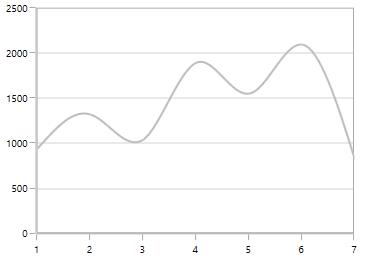NOTE

By default, Range is calculated between the minimum and maximum value of the data points.

### CategoryAxis

`CategoryAxis` is an indexed based axis that plots values based on the index of the data point collection. The points are equally spaced here. The following code example initializes the `CategoryAxis`.

``````<syncfusion:SfChart.PrimaryAxis>

<syncfusion:CategoryAxis>

</syncfusion:CategoryAxis>

</syncfusion:SfChart.PrimaryAxis>``````
``chart.PrimaryAxis = new CategoryAxis();``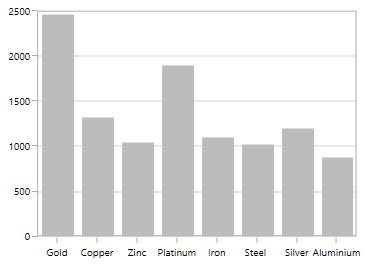LabelPlacement

In `CategoryAxis`, labels are placed based on tick lines using the `LabelPlacement` property. By default, the labels are placed `OnTicks`. The following code example demonstrates how to place the label between ticks in `CategoryAxis`.

``````<syncfusion:SfChart.PrimaryAxis>

<syncfusion:CategoryAxis LabelPlacement="BetweenTicks">

</syncfusion:CategoryAxis>

</syncfusion:SfChart.PrimaryAxis>``````
``````chart.PrimaryAxis = new CategoryAxis()
{

LabelPlacement = LabelPlacement.BetweenTicks

};``````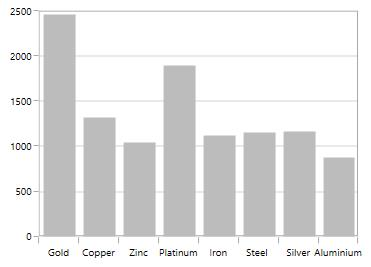IsIndexed

By default, `CategoryAxis` plots the values based on the index of the data points. However, by disabling the `IsIndexed` property of the `CategoryAxis`, it is possible to make the `CategoryAxis` plot the data points based on their data rather than their index value, and it is shown in the following code example.

``````<syncfusion:SfChart.PrimaryAxis>

<syncfusion:CategoryAxis IsIndexed="False"/>

</syncfusion:SfChart.PrimaryAxis>``````
``````chart.PrimaryAxis = new CategoryAxis()
{

IsIndexed = false,

};``````

The following screenshot illustrates the series with the IsIndexed value as False.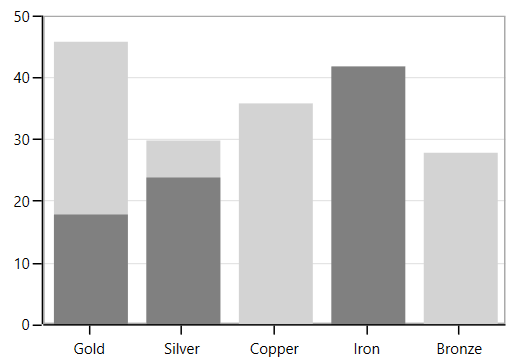By default, the IsIndexed property value is true, and it is shown in the following screenshot.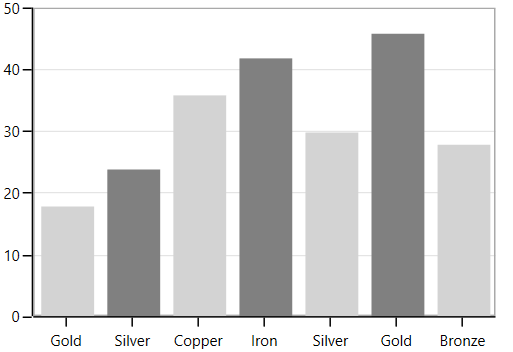NOTE

This feature is not applicable for AccumulationSeries, RadarSeries, and PolarSeries.

### DateTimeAxis

`DateTimeAxis` is used to plot DateTime values. The `DateTimeAxis` is widely used to make financial charts in places, like the Stock Market, where index plotting is done on a daily basis.

``````<syncfusion:SfChart.PrimaryAxis>

<syncfusion:DateTimeAxis LabelFormat="MMM-dd">

</syncfusion:DateTimeAxis>

</syncfusion:SfChart.PrimaryAxis>``````
``````chart.PrimaryAxis = new DateTimeAxis()
{

LabelFormat = "MMM-dd"

};``````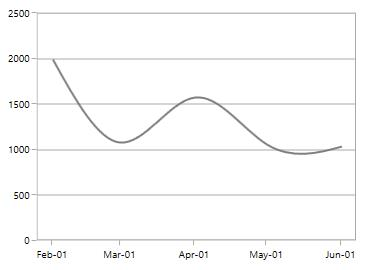Customizing the Range

`Minimum` and `Maximum` properties behavior is same as in NumericalAxis, but instead of setting numerical values, you have to set date-time values.

``````<syncfusion:SfChart.PrimaryAxis>

<syncfusion:DateTimeAxis Minimum="2015/01/10" Maximum="2015/07/01" LabelFormat="MMM-dd" IntervalType="Months" Interval="1">

</syncfusion:DateTimeAxis>

</syncfusion:SfChart.PrimaryAxis>``````
``````chart.PrimaryAxis = new DateTimeAxis()
{

Minimum = new DateTime(2015,01,10),

Maximum = new DateTime(2015,07,01),

LabelFormat = "MMM-dd",

IntervalType = DateTimeIntervalType.Months,

Interval = 1

};``````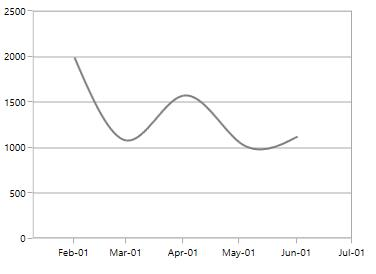`SfChart` provides support to plot only the business hours in DateTimeAxis. This support is enabled by setting the `EnableBusinessHours` property to true.

The following properties are used for business hour range calculation:

The following code sample demonstrates the business hours support in DateTimeAxis.

``````<syncfusion:SfChart.PrimaryAxis>

CloseTime="24" WorkingDays="Friday,Saturday,Sunday,Monday,Tuesday,Wednesday,Sunday">

</syncfusion:DateTimeAxis>

</syncfusion:SfChart.PrimaryAxis>``````
``````chart.PrimaryAxis = new DateTimeAxis()
{

OpenTime = 9,

CloseTime = 24,

WorkingDays = Day.Friday | Day.Saturday | Day.Sunday |
Day.Monday | Day.Tuesday| Day.Wednesday| Day.Sunday

};``````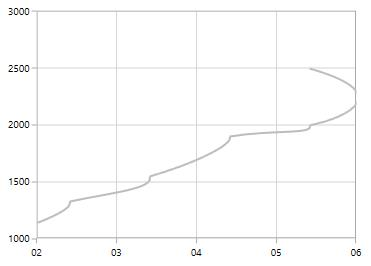## Inverting axis

Axis can be inverted using the `IsInversed` property. The default value of this property is `False`.

``````<chart:SfChart.SecondaryAxis>

<chart:NumericalAxis IsInversed="True"/>

</chart:SfChart.SecondaryAxis>``````
``this.Chart.SecondaryAxis.IsInversed = true;``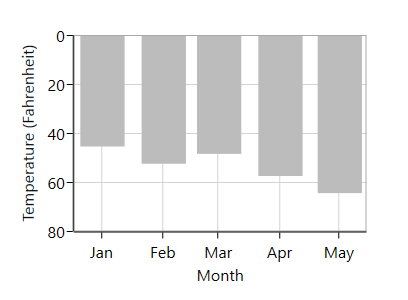## Customizing the Intervals

`ChartAxis` calculates the range and intervals automatically based on the data points. The axis range and interval can be defined using the `Minimum`, `Maximum`, and `Interval` properties.

NumericalAxis

The following code sample demonstrates the `Interval` customization in NumericalAxis.

``````<syncfusion:SfChart.SecondaryAxis>

<syncfusion:NumericalAxis Interval="250">

</syncfusion:NumericalAxis>

</syncfusion:SfChart.SecondaryAxis>``````
``````chart.SecondaryAxis = new NumericalAxis()
{

Interval = 250

};``````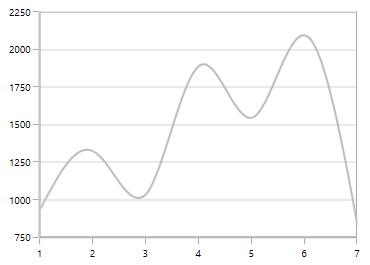CategoryAxis

The following code sample demonstrates the `Interval` customization in CategoryAxis.

``````<syncfusion:SfChart.PrimaryAxis>

<syncfusion:CategoryAxis Interval="2">

</syncfusion:CategoryAxis>

</syncfusion:SfChart.PrimaryAxis>``````
``````chart.PrimaryAxis = new CategoryAxis()
{

Interval = 2

};``````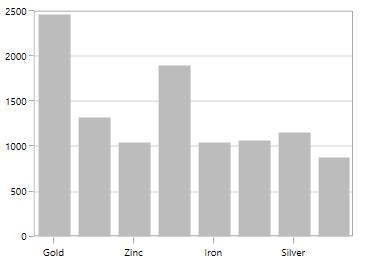DateTimeAxis

The DateTimeAxis `Interval` value corresponds to the type specified in the `IntervalType` property.

For instance, if the `Interval` is set to two and `IntervalType` is set to `Days`, the labels are plotted for every two days. The following are the options for `IntervalType` property.

• Auto
• Days
• Hours
• Milliseconds
• Minutes
• Months
• Seconds
• Years

The default `IntervalType` of a `DateTimeAxis` is Auto. It calculates the type and the interval automatically.

The following code snippet demonstrates the DateTimeAxis with a one-month interval.

``````<syncfusion:SfChart.PrimaryAxis>

<syncfusion:DateTimeAxis Interval="1" IntervalType="Months" LabelFormat="MMM-dd">

</syncfusion:DateTimeAxis>

</syncfusion:SfChart.PrimaryAxis>``````
``````chart.PrimaryAxis = new DateTimeAxis()
{

Interval = 1,

IntervalType = DateTimeIntervalType.Months,

LabelFormat = "MMM-dd"

};``````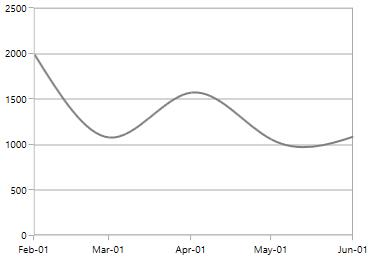DesiredIntervalsCount

`DesiredIntervalsCount` property is used to specify the count of the axis labels between the first and last label. The following sample code defines the `DesiredIntervalsCount` property.

``````<syncfusion:SfChart.SecondaryAxis>

<syncfusion:NumericalAxis DesiredIntervalsCount="7">

</syncfusion:NumericalAxis>

</syncfusion:SfChart.SecondaryAxis>``````
``````chart.SecondaryAxis = new NumericalAxis()
{

DesiredIntervalsCount = 7

};``````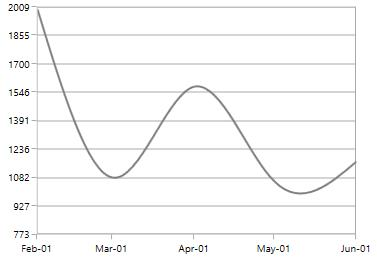Maximum Labels

`MaximumLabels` property defines the count of the axis labels in the 100 pixels.

``````<syncfusion:SfChart.SecondaryAxis>

<syncfusion:NumericalAxis MaximumLabels="2">

</syncfusion:NumericalAxis>

</syncfusion:SfChart.SecondaryAxis>

<syncfusion:ColumnSeries ItemsSource="{Binding Demands}" XBindingPath="Demand" YBindingPath="Year2010">

<syncfusion:ColumnSeries.YAxis>

<syncfusion:NumericalAxis MaximumLabels="2">

</syncfusion:NumericalAxis>

</syncfusion:ColumnSeries.YAxis>

</syncfusion:ColumnSeries>``````
``````chart.SecondaryAxis = new NumericalAxis()
{

MaximumLabels = 2

};

NumericalAxis axis = new NumericalAxis() { MaximumLabels = 2 };

ColumnSeries series = new ColumnSeries()
{

ItemsSource = new ViewModel().Demands,

XBindingPath = "Demand",

YBindingPath = "Year2010",

YAxis = axis

};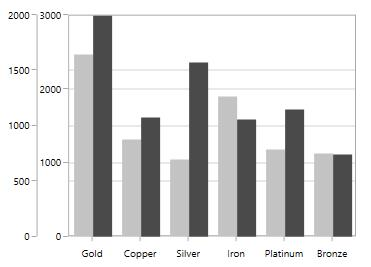## Apply Padding to the Range

The `NumericalAxis` and `DateTimeAxis` have a `RangePadding` property that can be used to add padding to the range of a chart’s axes.

The `RangePadding` types available in the `DateTimeAxis` are:

• Auto
• None
• Round
• RoundStart
• RoundEnd
• PrependInterval
• AppendInterval

Auto

By default, the date-time range padding is `Auto`.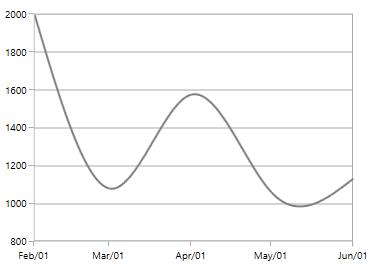When you use `RangePadding` for `DateTimeAxis`, the DateTime interval of the axis is added as padding, as shown in the following screenshot.

``````<syncfusion:SfChart.PrimaryAxis>

</syncfusion:DateTimeAxis>

</syncfusion:SfChart.PrimaryAxis>``````
``````chart.PrimaryAxis = new DateTimeAxis()
{

};``````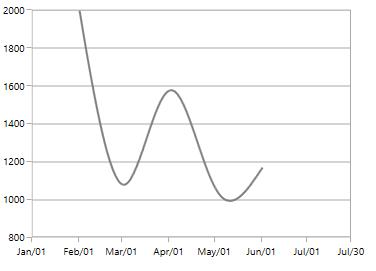Round

When `RangePadding` for `DateTimeAxis` is set to `Round`, the range of the chart axis is rounded off to the nearest possible DateTime value, as shown in the following screenshot.

``````<syncfusion:SfChart.PrimaryAxis>

</syncfusion:DateTimeAxis>

</syncfusion:SfChart.PrimaryAxis>``````
``````chart.PrimaryAxis = new DateTimeAxis()
{

};``````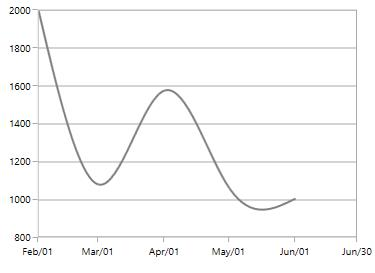None

When the `RangePadding` for a `DateTimeAxis` is `None`.

The following screenshot demonstrates a chart’s x-axis with `RangePadding` set to `None.`

``````<syncfusion:SfChart.PrimaryAxis>

</syncfusion:DateTimeAxis>

</syncfusion:SfChart.PrimaryAxis>``````
``````chart.PrimaryAxis = new DateTimeAxis()
{

};``````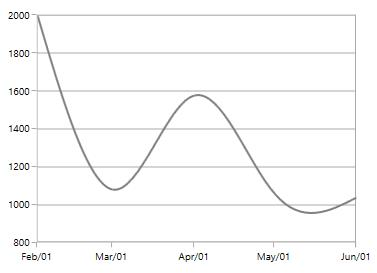RoundStart

When `RangePadding` for `DateTimeAxis` is set to `RoundStart`, the range of the chart axis is rounded in the start off to the nearest possible DateTime value, as shown in the following screenshot.

``````<syncfusion:SfChart.PrimaryAxis>

</syncfusion:DateTimeAxis>

</syncfusion:SfChart.PrimaryAxis>``````
``````chart.PrimaryAxis = new DateTimeAxis()
{

};``````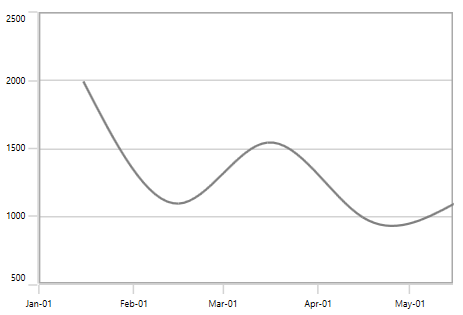RoundEnd

When `RangePadding` for `DateTimeAxis` is set to `RoundEnd`, the range of the chart axis is rounded in the end off to the nearest possible DateTime value, as shown in the following screenshot.

``````<syncfusion:SfChart.PrimaryAxis>

</syncfusion:DateTimeAxis>

</syncfusion:SfChart.PrimaryAxis>``````
``````chart.PrimaryAxis = new DateTimeAxis()
{

};``````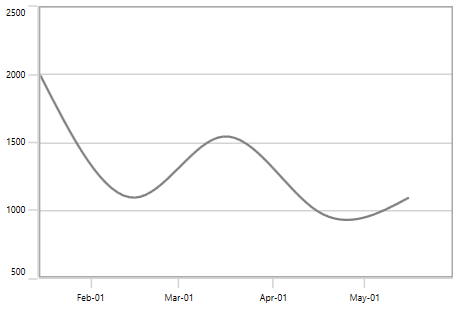PrependInterval

When `RangePadding` for `DateTimeAxis` is set to `PrependInterval`, the DateTime interval of the axis is added in the start as padding, as shown in the following screenshot.

``````<syncfusion:SfChart.PrimaryAxis>

</syncfusion:DateTimeAxis>

</syncfusion:SfChart.PrimaryAxis>``````
``````chart.PrimaryAxis = new DateTimeAxis()
{

};``````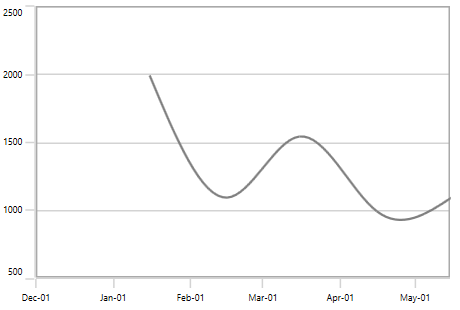AppendInterval

When `RangePadding` for `DateTimeAxis` is set to `AppendInterval`, the DateTime interval of the axis is added in the end as padding, as shown in the following screenshot.

``````<syncfusion:SfChart.PrimaryAxis>

</syncfusion:DateTimeAxis>

</syncfusion:SfChart.PrimaryAxis>``````
``````chart.PrimaryAxis = new DateTimeAxis()
{

};``````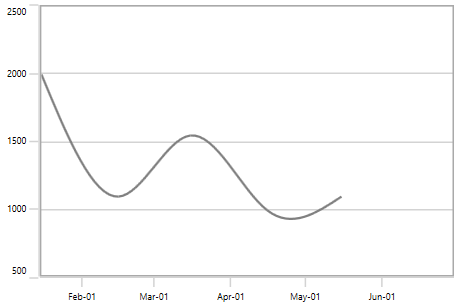The following types are available for `NumericalAxis`:

• None
• Normal
• Round
• Auto
• RoundStart
• RoundEnd
• PrependInterval
• AppendInterval

Round

By default, the default `RangePadding` value for `PrimaryAxis` is Auto and for `SecondaryAxis`, the default value is `Round`.

The following screenshot illustrates a chart’s y-axis with `RangePadding` set to `Round`.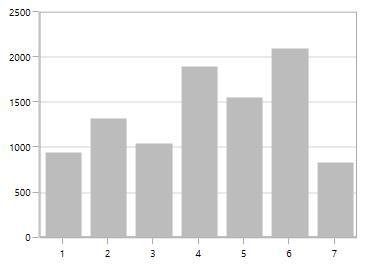Normal

`Normal` `RangePadding` for a `NumericalAxis` is used mostly for the y-axis to have padding based on the Range calculation.

The following screenshot illustrates a chart’s y-axis with `RangePadding` set to `Normal`.

``````<syncfusion:SfChart.PrimaryAxis>

</syncfusion:NumericalAxis>

</syncfusion:SfChart.PrimaryAxis>``````
``````chart.PrimaryAxis = new NumericalAxis()
{

};``````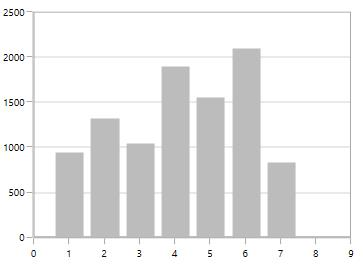If `RangePadding` for `NumericalAxis` is set to `Additional`, the interval of the axis is added as padding.

The following screenshot demonstrates a chart’s x-axis with `RangePadding` set to `Additional`.

``````<syncfusion:SfChart.PrimaryAxis>

</syncfusion:NumericalAxis>

</syncfusion:SfChart.PrimaryAxis>``````
``````chart.PrimaryAxis = new NumericalAxis()
{

};``````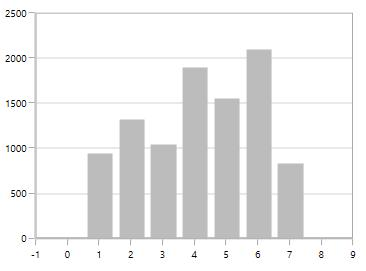None

The following screenshot demonstrates `RangePadding` as `None`, where no padding is applied for the axis.

``````<syncfusion:SfChart.PrimaryAxis>

</syncfusion:NumericalAxis>

</syncfusion:SfChart.PrimaryAxis>``````
``````chart.PrimaryAxis = new NumericalAxis()
{

};``````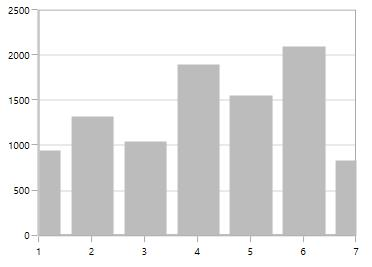RoundStart

If `RangePadding` for `NumericalAxis` is set to `RoundStart`, the chart axis range is rounded to the nearest possible value.

The following screenshot demonstrates a chart’s x-axis with `RangePadding` set to `RoundStart`.

``````<syncfusion:SfChart.PrimaryAxis>

</syncfusion:NumericalAxis>

</syncfusion:SfChart.PrimaryAxis>``````
``````chart.PrimaryAxis = new NumericalAxis()
{

};``````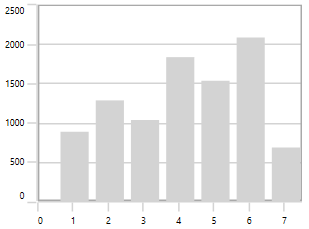RoundEnd

If `RangePadding` for `NumericalAxis` is set to `RoundEnd`, the chart axis range is rounded to the nearest possible value at the end.

The following screenshot demonstrates a chart’s x-axis with `RangePadding` set to `RoundEnd`.

``````<syncfusion:SfChart.PrimaryAxis>

</syncfusion:NumericalAxis>

</syncfusion:SfChart.PrimaryAxis>``````
``````chart.PrimaryAxis = new NumericalAxis()
{

};``````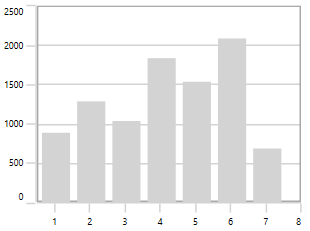PrependInterval

If `RangePadding` for `NumericalAxis` is set to `PrependInterval`, the interval of the axis is added in the start as padding.

The following screenshot demonstrates a chart’s x-axis with `RangePadding` set to `PrependInterval`.

``````<syncfusion:SfChart.PrimaryAxis>

</syncfusion:NumericalAxis>

</syncfusion:SfChart.PrimaryAxis>``````
``````chart.PrimaryAxis = new NumericalAxis()
{

};``````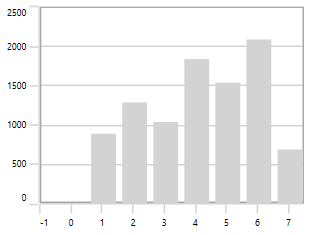AppendInterval

If `RangePadding` for `NumericalAxis` is set to `AppendInterval`, the interval of the axis is added in the end as padding.

The following screenshot demonstrates a chart’s x-axis with `RangePadding` set to `AppendInterval`.

``````<syncfusion:SfChart.PrimaryAxis>

</syncfusion:NumericalAxis>

</syncfusion:SfChart.PrimaryAxis>``````
``````chart.PrimaryAxis = new NumericalAxis()
{

};``````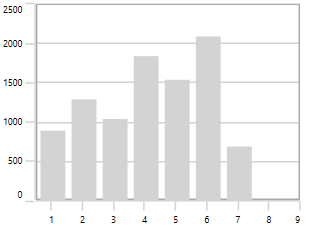## Applying Padding to the Axis

`PlotOffset` property is used to provide padding to the axis. The following code sample demonstrates the padding applied to both x and y axes.

``````<syncfusion:SfChart.PrimaryAxis>

<syncfusion:NumericalAxis PlotOffset="30">

</syncfusion:NumericalAxis>

</syncfusion:SfChart.PrimaryAxis>

<syncfusion:SfChart.SecondaryAxis>

<syncfusion:NumericalAxis PlotOffset="30">

</syncfusion:NumericalAxis>

</syncfusion:SfChart.SecondaryAxis>``````
``````chart.PrimaryAxis = new NumericalAxis()
{

PlotOffset = 30

};

chart.SecondaryAxis = new NumericalAxis()
{

PlotOffset = 30

};``````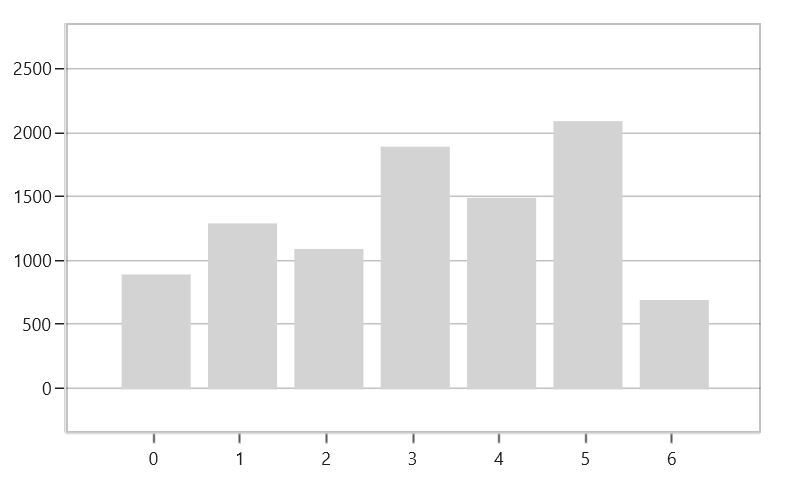### PlotOffsetStart

`PlotOffsetStart` property is used to provide padding to the axis at start position. The following code sample demonstrates the padding applied to start position for both x and y axes.

``````<syncfusion:SfChart.PrimaryAxis>

<syncfusion:NumericalAxis PlotOffsetStart="30">

</syncfusion:NumericalAxis>

</syncfusion:SfChart.PrimaryAxis>

<syncfusion:SfChart.SecondaryAxis>

<syncfusion:NumericalAxis PlotOffsetStart="30">

</syncfusion:NumericalAxis>

</syncfusion:SfChart.SecondaryAxis>``````
``````chart.PrimaryAxis = new NumericalAxis()
{

PlotOffsetStart = 30

};

chart.SecondaryAxis = new NumericalAxis()
{

PlotOffsetStart = 30

};``````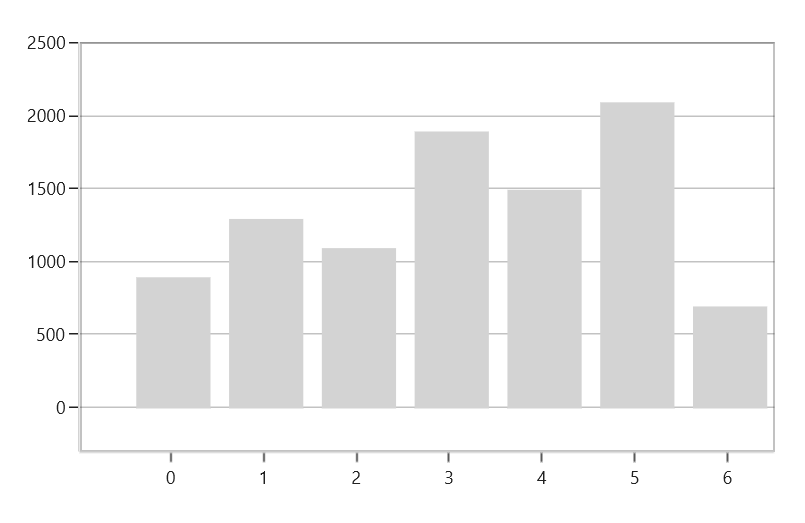### PlotOffsetEnd

`PlotOffsetEnd` property is used to provide padding to the axis at end position. The following code sample demonstrates the padding applied to end position for both x and y axes.

``````<syncfusion:SfChart.PrimaryAxis>

<syncfusion:NumericalAxis PlotOffsetEnd="30">

</syncfusion:NumericalAxis>

</syncfusion:SfChart.PrimaryAxis>

<syncfusion:SfChart.SecondaryAxis>

<syncfusion:NumericalAxis PlotOffsetEnd="30">

</syncfusion:NumericalAxis>

</syncfusion:SfChart.SecondaryAxis>``````
``````chart.PrimaryAxis = new NumericalAxis()
{

PlotOffsetEnd = 30

};

chart.SecondaryAxis = new NumericalAxis()
{

PlotOffsetEnd = 30

};``````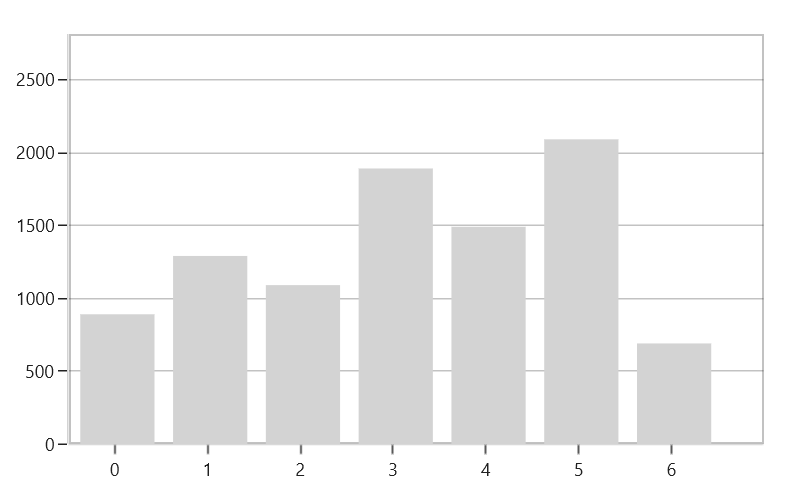## AutoScrollingDelta

`AutoScrollingDelta` is used to ensure whether the specified range of data is always visible in the chart. It always shows the recently added data points at the end, and the scrolling will be reset to the end of the range whenever a new point is added.

### AutoScrollingDeltaType

In `DateTimeAxis`, you can apply auto scrolling delta value in `Years`, `Months`, `Days`, `Hours`, `Minutes`, `Seconds`, and `Milliseconds` by setting the `AutoScrollingDeltaType` property. The default value of this property is `Auto`, and the delta will be calculated automatically based on range.

``````<chart:SfChart.PrimaryAxis>

<chart:DateTimeAxis AutoScrollingDelta = "3" AutoScrollingDeltaType = "Days">

</chart:SfChart.PrimaryAxis>``````
``````chart.PrimaryAxis = new DateTimeAxis()
{
AutoScrollingDelta = 3,

AutoScrollingDeltaType = DateTimeIntervalType.Days
};``````

### AutoScrollingMode

The `AutoScrollingMode` property is used to determine whether the axis should be scrolled from the start position or end position. The default value of this property is `End`.

``````<chart:SfChart.PrimaryAxis>

<chart:DateTimeAxis AutoScrollingDelta = "3" AutoScrollingMode = "Start">

</chart:SfChart.PrimaryAxis>``````
``````chart.PrimaryAxis = new DateTimeAxis()
{
AutoScrollingDelta = 3,

AutoScrollingMode = ChartAutoScrollingMode.Start
};``````

## Auto Interval Calculation on Zooming

`EnableAutoIntervalOnZooming` property is used to maintain the interval, even if it is in a zooming state, only if we set the interval to the axis. The default value of this property is true. While zooming based on the auto-range padding the interval will be calculated.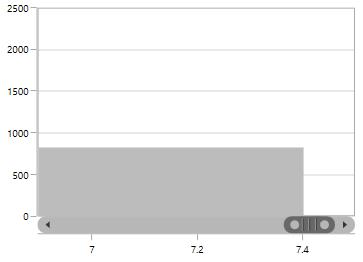If you set `EnableAutoIntervalOnZooming` as False, the intervals will be calculated on the interval based on the axis while zooming.

``````<syncfusion:SfChart.PrimaryAxis>

<syncfusion:NumericalAxis Interval="1" EnableAutoIntervalOnZooming="False">

</syncfusion:NumericalAxis>

</syncfusion:SfChart.PrimaryAxis>``````
``````chart.PrimaryAxis = new NumericalAxis()
{

EnableAutoIntervalOnZooming = false,

Interval = 1

};``````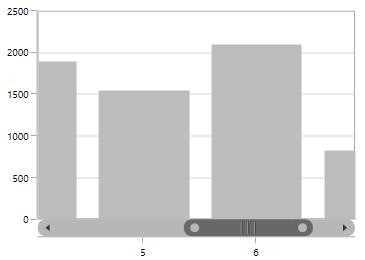## Multiple Axes

SfChart provides a way to arrange multiple series inside the same chart area, giving the chart more space than the x-axis and y-axis. These axes can be arranged in a stacking order or in a side by side pattern.

By default, all the series are plotted based on primary and secondary axis. You can add more axes by adding additional axis to the series. There are two properties `XAxis` and `YAxis` in all the series type, which is used to provide Multiple axes support, except `CircularSeries`, `PyramidSeries`, and `FunnelSeries`.

``````<syncfusion:ColumnSeries ItemsSource="{Binding Demands}"
XBindingPath="Demand" YBindingPath="Year2011">

</syncfusion:ColumnSeries>

<syncfusion:LineSeries  ItemsSource="{Binding Demands}"
XBindingPath="Date"  YBindingPath="Year2011">

<syncfusion:LineSeries.XAxis>

<syncfusion:DateTimeAxis />

</syncfusion:LineSeries.XAxis>

<syncfusion:LineSeries.YAxis>

<syncfusion:NumericalAxis/>

</syncfusion:LineSeries.YAxis>

</syncfusion:LineSeries>``````
``````ColumnSeries series1 = new ColumnSeries()
{

ItemsSource = new ViewModel().Demands,

XBindingPath = "Demand",

YBindingPath = "Year2011"

};

LineSeries series2 = new LineSeries()
{

ItemsSource = new ViewModel().Demands,

XBindingPath = "Date",

YBindingPath = "Year2011",

};

series2.XAxis = new DateTimeAxis()
{

};

series2.YAxis = new NumericalAxis()
{

};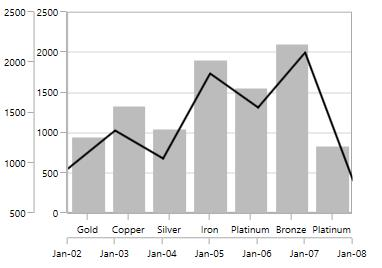In the above screenshot, the LineSeries is plotted based on additional X and Y axes, and ColumnSeries (or remaining series) is plotted based on the primary and secondary axes.

## Events

### ActualRangeChanged

The `ActualRangeChanged` event occurs when an axis range is changed. This argument contains the following information.

### LabelCreated

The `LabelCreated` event occurs when the axis label is created. This argument contains `AxisLabel` of `ChartAxisLabel`, which has the following properties:

### AxisBoundsChanged

The `AxisBoundsChanged` event occurs when the bounds of the axis are changed. This argument contains the following information.

### LabelClicked

The `LabelClicked` event is triggered when labels are clicked. The argument contains `Label` of `ChartAxisLabel`, which has the following properties.